# Difference between revisions of "Finite-increments formula"

Lagrange finite-increments formula

A formula expressing the increment of a function in terms of the value of its derivative at an intermediate point. If a functionis continuous on an intervalon the real axis and is differentiable at the interior points of it, thenThe finite-increments formula can also be written in the form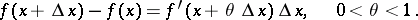The geometric meaning of the finite-increments formula is: Given the chord of the graph of the functionwith end points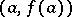,, then there exists a point,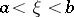, such that the tangent to the graph of the function at the pointis parallel to the chord (see Fig.).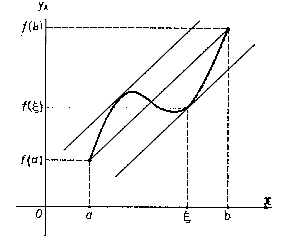Figure: f040300a

The finite-increments formula can be generalized to functions of several variables: If a functionis differentiable at each point of a convex domainin an-dimensional Euclidean space, then there exists for each pair of points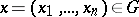,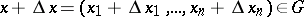a point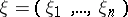lying on the segment joiningand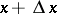and such that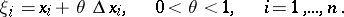This formula is usually called the mean-value theorem (for derivatives). It is a statement for real-valued functions only; consider, e.g.,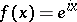.